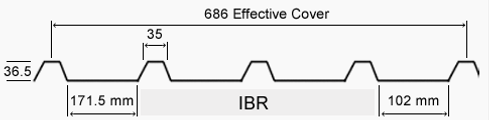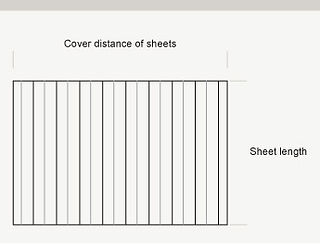top of page

## Profiles & Measuring## Square fluted profile with an effective covering width of 686mmCalculating the number of IBR sheets you'll need can be done simply by dividing the distance your IBR sheets need to cover by 686 mm.

Let's take for example the cover distance 4116mm divided by 686mm = 6 sheets

Using this formula and for example your cover distance is 8000 mm then divided by 686 mm this will equal 11.6 sheets, then you'll round up the number of sheets to 12.## S-rib profile with an effective covering width of 762mmCalculating the number of corrugated sheets you'll need can be done simply by dividing the distance your corrugated sheets need to cover by 762 mm.

Let's take for example the cover distance 4572mm divided by 762mm = 6 sheets

Using this formula and for example your cover distance is 5000 mm then divided by 762 mm this will equal 6.56 sheets, then you'll round up the number of sheets to 7.bottom of page